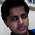### Divisibility Problem

Source:
Posted by JDGM (James Miles) in comments on http://www.puzzletweeter.com ( A fanstastic blog by Gowtham Kumar, PhD Student, Stanford, IITM Alumnus)

Problem:
Prove that from the numbers 1 to 200 we cannot pick 101 of them such that none divide any other.

Update (24 June 2014):

1.Express each number 1 to 200 as 2^k * p.
Now p can be 1,3,5,....199. So there are 100 distinct p. So by PHP if we choose 101 number then two number will have same p.
Let these number are a=2^k1 * p and b=2^k2 * p.
So depending on k1>k2 or k1<k2 b will divide a and vice-versa.

1.2.take 100 series
1. (1,2,4,8,...128)
2. (3,6,12,...192)
3. (5,10,20,...160)
.
.
.
100. (199)

There are all 200 numbers in them, and if you pick any 2 numbers from any series, they'll be divisible by each other by factor of power of 2.

By pigeon-hole principle, we can't have 101 number without one being divisible by other.

1.Thanks Ameya

3.Pigeon hole principle.

4.This is due to Paul Erdos.

We may write each number in the form 2^k*m where m is an odd integer. There are at most 100 possible values for m. Thus by the pigeonhole principle, we must have two with the same m value and one even part will divide the other.

1.Thanks Wei.

5.Suppose at first we pick numbers 101 to 200. Let's call it set S.So we have 100 numbers in set S. In order to increase the number of numbers in set S such that none divides any other we have to remove one number,lets say N from set S and add two or more numbers from 67-100 in set S such that these numbers divide N. But no such numbers exist. So the maximum numbers that can be picked with the constraint given is 100.

1.Just because it cannot be constructed by the method you showed, does not mean it does not exist. What is there are x elements < 100 and not 1?

6.1.Awesome :-) Thanks

7.Suppose it is possible.
Then, I divide 1-200 into two groups: 1-100;101-200. Each having 100 each in the group.

Now as it was possible, thus assume that in the possible case you have k numbers chosen from group 1-100 and 101-k from the group 101-200. (As we have to choose 101 such numbers)

Now, note that with every number in the group 1-100, there corresponds a number in 101-200 that cannot be chosen. This is because, say we chose 62 from 1-100. But then 62*2=124 cannot be chosen from 101-200.

Therefore there would be at least k such numbers in the group 101-200 that can't be chosen. Therefore we only have 100-k possibilities left in the group 1-100, while we have to choose 101-k. (Being greater that 100-k)

This should be it I think.

1.Why would all the k numbers to be neglected in 101-200 set would be distinct ?

2.Yep. Agree with Gibraltar point. Unknown, your solution is not correct. Thanks for the attempt though.